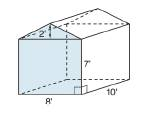Chapter 9.1, Problem 29E### Elementary Geometry for College St...

6th Edition
Daniel C. Alexander + 1 other
ISBN: 9781285195698

#### Solutions

Chapter
Section### Elementary Geometry for College St...

6th Edition
Daniel C. Alexander + 1 other
ISBN: 9781285195698
Textbook Problem
1 views

# A storage shed is in the shape of a pentagonal prism. The front represents one of its two pentagonal bases. What is the storage capacity (volume) of its interior?To determine

To find:

The storage capacity (volume) of a storage shed in the shape of a pentagonal prism.

Explanation

The volume of any solid is the region of space occupied by it and in general the volume of a solid is given by the product of its base area and altitude.

For a regular solid prism with base area B and altitude h, the volume is given by the formula

V=Bh.

Calculation:

Given,

A pentagonal prism with an irregular pentagonal base.

The pentagonal base has two sections, one a rectangular section of dimension 8 in.  × 7 in. and a triangular section of base 8 in and height 2 in.

The height of the shed is 10 in.

The capacity (volume) of the storage shed (pentagonal prism) with base area B and height ‘h’ is given by the formula

V=Bh

Let us calculate the base area ‘B’ of the pentagonal prism.

Since, the base is not a regular pentagonal, its area is given by

B= Area of the rectangular part of the base + Area of the triangular part of the base

### Still sussing out bartleby?

Check out a sample textbook solution.

See a sample solution

#### The Solution to Your Study Problems

Bartleby provides explanations to thousands of textbook problems written by our experts, many with advanced degrees!

Get Started

#### Find more solutions based on key concepts